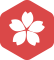Beanocean

MATLAB中批量导入.mat文件（每个文件变量

Matlab读写.mat数据

【20140419】【MatlabMatlab中.M文件和.mat文件的区别以及.mat文件的生成和调用方法

.m文件和.mat文件的区别 1. .M文件 是指Matlab中保存代码的文件；...save('data.mat','a','b','c') %假如有三个变量'a', 'b', 'c'，要将它们保存在'data'数据文件中。 2. 调用数据文件：用loa...

Matlab一个矩阵保存在.mat文件中，或读取.mat文件

MATLAB中修改mat文件中变量的名称

matlabMatlab中实现数据以.mat文件形式的存储和读取

save(filename) %将当前工作区的所有变量保存在 MATLAB® 格式的二进制文件MAT 文件）filename 。 save(filename,variables) %仅保存 variables 指定的结构体数组的变量或字段。 官方save函数介绍：...

Matlab将多个.mat文件内的数据行拼接到一个.mat文件

namelist = dir(‘C:\Users\王浩\Desktop\new2000\原数据\af200*.mat’); len = length(namelist); A=[]; for i = 1:len file_name{i}=namelist(i).name; load(file_name{i}); temp=importdata(file_name{i}); A=[A;...

MATLAB修改.mat文件中的内容并保存

data.mat中存储的变量名未必就是data load 完后检查一下workspace 里的矩阵的名字是什么？或者输入 whos 命令查看一下。然后用正确的变量名来访问你的数据 于是我们再去加载数据，注意采用这种方式即可 ...

matlab文件中文件重命名及将多个文件保存为一个.mat文件

#使用 points=importdata(a); #其中a是已经存放在当前文件夹的.mat #最好不要使用points=load(a); 此时导入的a是一个struct 类型的数据，无法进行正常的调用

MATLAB循环读取不同路径下的.mat文件

Matlab中.mat文件的生成、读取、调用方法 .mat文件Matlab的数据存储的标准格式，它是标准的二进制文件，还可以以ASCII码形式保存和加载，在Matlab中打开显示类似于Excel表格。 .mat文件本质：标准的二进制文件 ...

matlab基础笔记（二）mat文件与m文件的区别及应用

.M文件是保存一段代码的文件，类似于C语言一个函数体； 这也是MATLAB中最常见的文件保存格式之一 .matmatlab的数据存储的标准格式。也就是你操作产生的数据的一个集合包，可以把一次处理的结果保存，供下一...

matlab下的.mat数据存储为txt文件(实例讲解)

matlab下的.mat数据存储为txt文件【实例讲解】 已知一个SINS_DATA.mat数据文件，其数据内容如下图所示， 上图第7～12列的数据代表的是IMU的陀螺和加计数据，现在要将它们分离出去，单独存到一个txt文件中，代码...

save(filename) %将当前工作区的所有变量保存在 MATLAB®格式的二进制文件MAT 文件）filename。 save(filename,variables) %仅保存variables指定的结构体数组的变量或字段。 MATLAB示例 A=[1 2 3 ]; B=...

MATLAB 生成.mat 文件及读取

MATLAB的.mat文件与txt文件的相互转换（mat转txt，数据格式设置）

%加载txt文件，加载成功后，在Workspace出现与该txt文件同名的变量。 %注意：若txt文件名有“-”字符，则Workspace中变量相应字符变为“_” Save('路径\xx.mat','变量名') 例： [plain] ...

Matlab--存储和加载.mat文件形式的数据

%指定文件夹存储工作空间所有变量 save(‘D:\Matlab\Project\filename.mat'); %当前文件夹存储工作空间所有变量 save filename; %指定文件夹存储工作空间指定的变量 save('D:\Matlab\Project\filename....

matlab读取txt到.mat

C++读写matlab数据.mat文件

python 调用matlab函数，加载.mat文件 各类方式说明、填坑

matlab GUI界面设计 点击按钮加载.mat数据的所有变量到工作空间